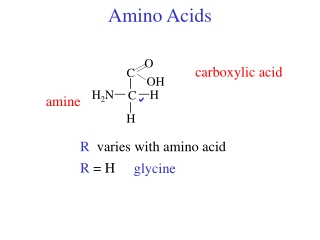DownloadDownload PresentationAmino Acids

# Amino Acids

Télécharger la présentation## Amino Acids

- - - - - - - - - - - - - - - - - - - - - - - - - - - E N D - - - - - - - - - - - - - - - - - - - - - - - - - - -
##### Presentation Transcript

1. O C OH Amino Acids carboxylic acid H2N H C amine  H R R varies with amino acid R = H glycine

2. -CH-CH3 CH3 -CH2-CH-CH3 CH3 -CH-CH2-CH3 CH3 Nonpolar R-groups -H glycine alanine -CH3 valine proline 2o amine leucine phenylalanine isoleucine methionine -CH2-CH2-S-CH3

3. -CH2-C-NH2 -CH2-C-NH2 O O -CH-CH3 -CH2 OH Polar R-groups serine asparagine -CH2-OH threonine glutamine tyrosine tryptophan cysteine -CH2-SH

4. O = -CH2-CH2-C OH O = -CH2-C OH Acidic R-groups glutamic acid aspartic acid

5. -CH2-CH2-CH2-NH-C-NH2 = NH Basic R-groups lysine -CH2-CH2-CH2-CH2-NH2 arginine histadine

6. C H H2N R O C OH Amino acids O- H3N+ H R glycine non-chiral all other -amino acids in proteins L-enantiomers zwitterion at neutral pH (pH = 7.0) very high b.p. (> 200oC) very soluble in water

7. C H C H H3N+ H2N C H H2N R R R O O O C C C O- OH OH acid-base chemistry O- H3N+ low pH neutral pH high pH amine protonated amine and c.a. deprotonated amine and c.a. protonated c.a. deprotonated no net charge negative charge positive charge pH = pHI isoelectric point amino acids diprotic acids 2 pKa

8. H3N+ C H R O C OH Titration of an amino acid alanine R = CH3 pKa1 = 2.34 pKa2 = 9.69 4.57 x 10-3 Ka1 = 10-2.34 = CH3 Ka1 = [H+][A-] [H+] [A-] [HA] 0.1 M [HA] initial 0 0 0.1 change +x +x -x equil. +x +x 4.57 x 10-3 = x2 0.1-x 0.1 - x • pH = 1.87 [H+] X = 2.14 x 10-2 =

9. 9.0 8.0 7.0 6.0 pH 5.0 4.0 3.0 2.0 1.0 0 equivalents of OH- net charge +1 ×

10. net charge 9.0 8.0 7.0 6.0 pH 5.0 4.0 3.0 2.0 × 1.0 0 equivalents of OH- +1 +1/2 0.05 M pKa1 = 2.34 0.05 M 0.05 M × pH = pKa + log [A-] [HA] 0.05 M 1/2 pH = pKa = 2.34

11. pKa2 = 9.69 9.0 8.0 7.0 6.0 pH 5.0 4.0 3.0 × 2.0 × 1.0 0 equivalents of OH- net charge at equivalence point: +1 +1/2 0 pKa1 = 2.34 × isoelectric point pKa1 + pKa2 pH = 2 pH = (2.34 + 9.69)/2 1 1/2 pH = pHI = 6.02

12. net charge 9.0 8.0 7.0 6.0 pH 5.0 4.0 3.0 2.0 × 1.0 0 equivalents of OH- at 2nd half-way point: +1 +1/2 0 -1/2 -1 × × pKa2 = 9.69 × pH = pKa2 = 9.69 1 1/2 3/2

13. resonance structure amides dipeptide glycine alanine Ala-Gly +H2O

14. H H H _ _ _ = = = O O O R R R _ _ _ Proteins “backbone” _ H N1- C1- C1- N2- C2- C2- N3- C3- C3- OH peptide bonds C-terminal residue N-terminal residue biological activity = structure 4 levels protein structure order of the amino acids Primary structure =

15. H H H _ _ _ _ H N1- C1- C1- N2- C2- C2- N3- C3- C3- OH = = = O O O R R R _ _ _ Secondary structure hydrogen bonding backbone groups H-bond donors H-bond acceptors -helix Two main secondary structures: -sheet

16. H C N = O C = O Alpha helix Every C=O bonded to N-H 4 residues away forms a helix core is backbone R-groups outside 3.6 amino acids per turn proline no H-bonding breaks helix

17. Beta sheet Every C=O bonded to N-H far apart in 1o structure on different chains peptide chains extended side-by-side maximal H-bonding for anti-parallel chains small R-groups above and below the sheet if not -helix or -sheet random coil

18. H3N+ C H O = CH2CH2C R OH O C OH glutamic acid R = - CH2CH2COOH (-COOH) pKa1 = 3.20 pKa2 = 4.25 (R-COOH) pKa3 = 9.67 (-NH3) It will take ___ equivalents to titrate glutamic acid 3 1st group 2nd group 3rd group

19. O -2 +1 0 -1 C 12 OH H3N+ H C = O CH2CH2C 10 × OH 8 pKa1 = 3.20 × pH pKa2 = 4.25 pHI = 3.7 pKa3 = 9.67 × 4 × × = 3.7 3.2 + 4.25 2 2 = 7.0 4.25 + 9.67 2 1 2 3 equivalents OH-